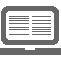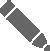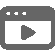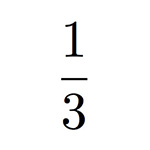CoursExercicesQuizzVidéos# Multiplications de fractions Correction exercice 4ème

• 6/3 × 2/5 = 6 × 2/3 × 5 = 12/15

• 13/5 × 7/8 = 13 × 7/5 × 8 = 91/40

• 12/7 × 4/9 = 4/7 × 4/3 = 4 × 4/7 × 3 = 16/21

• 14/5 × 12/11 = 14 × 12/5 × 11 = 168/55

• 3/4 × 8/6 = 1/1 × 2/2 =1 × 1 = 1

 Multiplications de fractions - Exercices de maths 4ème - Multiplications de fractions : 3/5 (12 avis) Donnez votre avis sur cet exercice. Excellent Très bien Bien Moyen MauvaisApollineda78 • il y a 916 jours. FACILE

Identifie-toi pour voir plus de contenu.